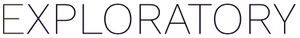# How to show the max value in a certain column as a text in Note

#1

Suppose you have sales data. Each row represents an order. Now, you want to show the max sales amount per order as a text in the note like “The max sales amount per order is \$xxxx”. Here is how.

First, check the data frame name and column name you want to show.

Then, open a note and write an R expression to get the max value. You can refer a column in the following syntax.

``````(data frame name)\$(column name)
``````

In this case, it will be

``````Global_Sales\$Sales
``````

Once you are done with the R expression, surround the expression with “`r” and “`” like following.

```````r max(Global_Sales\$Sales)`
``````

This portion will be evaluated and replaced with the output in the final document. You can hit the Refresh button to see the output.

#2

What expression would you use if you wanted to output “John Doe had the max sales amount per order (\$4912.59).”? I have a similar use case.

Total number of weekdays in a month?
#3

This related DM reply (from Kei) helped me so much!

Yes. You can use the following notation to access the value.

``````(dataframe name)\$(value column name)[[(key column name)=="(key value)"]]
``````

I basically added “max(dataframe name\$value column name)” after the “==” and it worked for my situation.